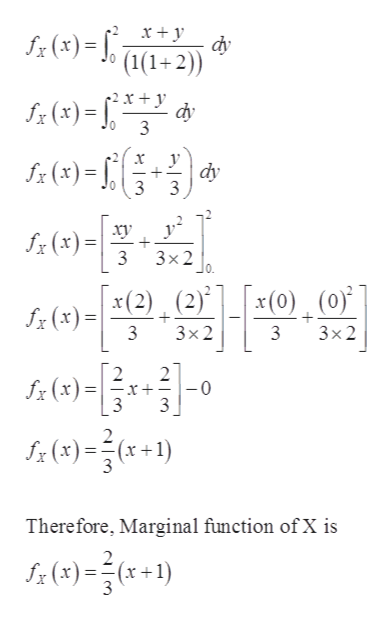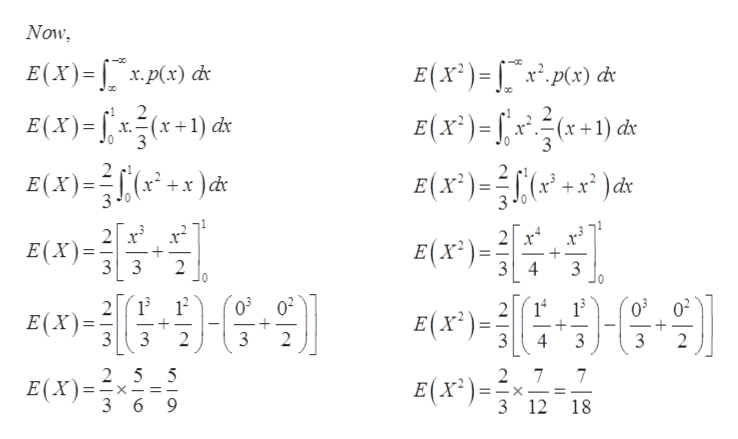# f (x, y) =(x+y)/(1(1 + 2)) is a joint probability density function over the range 0 < x < 1 and 0 < y < 2. Find V (X). Please report your answer to 3 decimal places.

Question
80 views

f (x, y) =(x+y)/(1(1 + 2)) is a joint probability density function over the range 0 < x < 1 and 0 < y < 2. Find V (X). Please report your answer to 3 decimal places.

check_circle

Step 1

In this question we have the joint probability density function of x and y, given below and for which we have to find the variance of X.

Step 2

Here, we have calculated the Marginal function of Xhelp_outlineImage Transcriptionclosedy f(x)=J ((1+2) (4) = dy 3 0 dy (x)= 3 - - 0 f (x)= 3x2 f(x)2)(2)L (0). (0)* ° 3 3x2 3 3x2 2 x(x)f 3 3 - 2 (x) Therefore, Marginal function of X is 3 fullscreen
Step 3

Here, We have calculated the value of ...help_outlineImage TranscriptioncloseNow E(x)x.p(x) d x E(x)=(1) E(X)- ) 2 x2=(x+1) d x.(x dx x2)d: +xd 3 2 x E(X) E(x*)= 34 33 2 0 3 03 02 14 02 티(X')-3 E(X) 3 2 3 2 4 3 3 2 2 7 E(x*) 2 5 5 7 E(X)=x 3 6 9 3 12 18 X fullscreen

### Want to see the full answer?

See Solution

#### Want to see this answer and more?

Solutions are written by subject experts who are available 24/7. Questions are typically answered within 1 hour.*

See Solution
*Response times may vary by subject and question.
Tagged in

### Probability